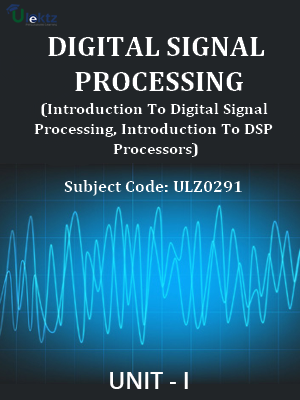•My WalletMy Order
•My Profile
•My Connections
•My Books
•My Videos
•My Tests
•My Calender
•My Messages
•My Shopping Cart
•My Orders
•Account Settings
•Help

# Book Details# Digital Signal Processing (Introduction To Digital Signal Processing, Introduction To DSP Processors)

 Course Code : ULZ0291 Author : uLektz University : General for All University Regulation : 2017 Categories : Electronics & Communication Format :ePUB3 (DRM Protected) Type : eBook

FREE

Description :Digital Signal Processing (Introduction To Digital Signal Processing, Introduction To DSP Processors) of ULZ0291 covers the latest syllabus prescribed by General for All University for regulation 2017. Author: uLektz, Published by uLektz Learning Solutions Private Limited.

Note : No printed book. Only ebook. Access eBook using uLektz apps for Android, iOS and Windows Desktop PC.

##### Topics
###### UNIT I INTRODUCTION

1.1 Introduction to Digital Signal Processing

1.2 Discrete time signals & sequences, Linear shift invariant systems, Stability and causality

1.3 Linear constant coefficient difference equations, Frequency domain representation of discrete time signals and systems

###### UNIT II DISCRETE FOURIER SERIES & FOURIER TRANSFORMS

2.1 Properties of discrete Fourier series, DFS representation of periodic sequences

2.2 Discrete Fourier transforms: Properties of DFT, Linear convolution of sequences using DFT, Computation of DFT

2.3 Fast Fourier transforms (FFT), Radix-2 decimation in time and decimation in frequency FFT Algorithms, Inverse FFT

###### UNIT III REALIZATION OF DIGITAL FILTERS

3.1 Review of Z-transforms, Applications of Z – transforms, solution of difference equations - digital filters, Block diagram representation of linear constant-coefficient difference equations

3.2 Basic structures of IIR systems, Transposed forms

3.3 Basic structures of FIR systems, System function

###### UNIT IV IIR & FIR DIGITAL FILTERS

4.1 Analog filter approximations – Butter worth and Chebyshev, Design of IIR Digital filters from analog filters, Design Examples: Analog-Digital transformations

4.2 Characteristics of FIR Digital Filter, Frequency response, Design of FIR Digital Filters using Window Techniques

4.3 Frequency Sampling technique, Comparison of IIR & FIR filters

###### UNIT V MULTIRATE DIGITAL SIGNAL PROCESSING

5.1 Decimation

5.2 Interpolation

5.3 Sampling rate conversion, Implementation of sampling rate conversion

###### UNIT VI INTRODUCTION TO DSP PROCESSORS

6.1 Introduction to programmable DSPs: Multiplier and Multiplier Accumulator (MAC), Modified Bus Structures and Memory Access schemes in DSPs, Multiple access memory, multiport memory

6.2 VLIW architecture, Pipelining, Special addressing modes, On-Chip Peripherals

6.3 Architecture of TMS 320C5X- Introduction, Bus Structure, Central Arithmetic Logic Unit, Auxiliary Register, Index Register, Block Move Address Register, Parallel Logic Unit, Memory mapped registers, program controller, Some flags in the status registers, On- chip registers, On-chip peripherals